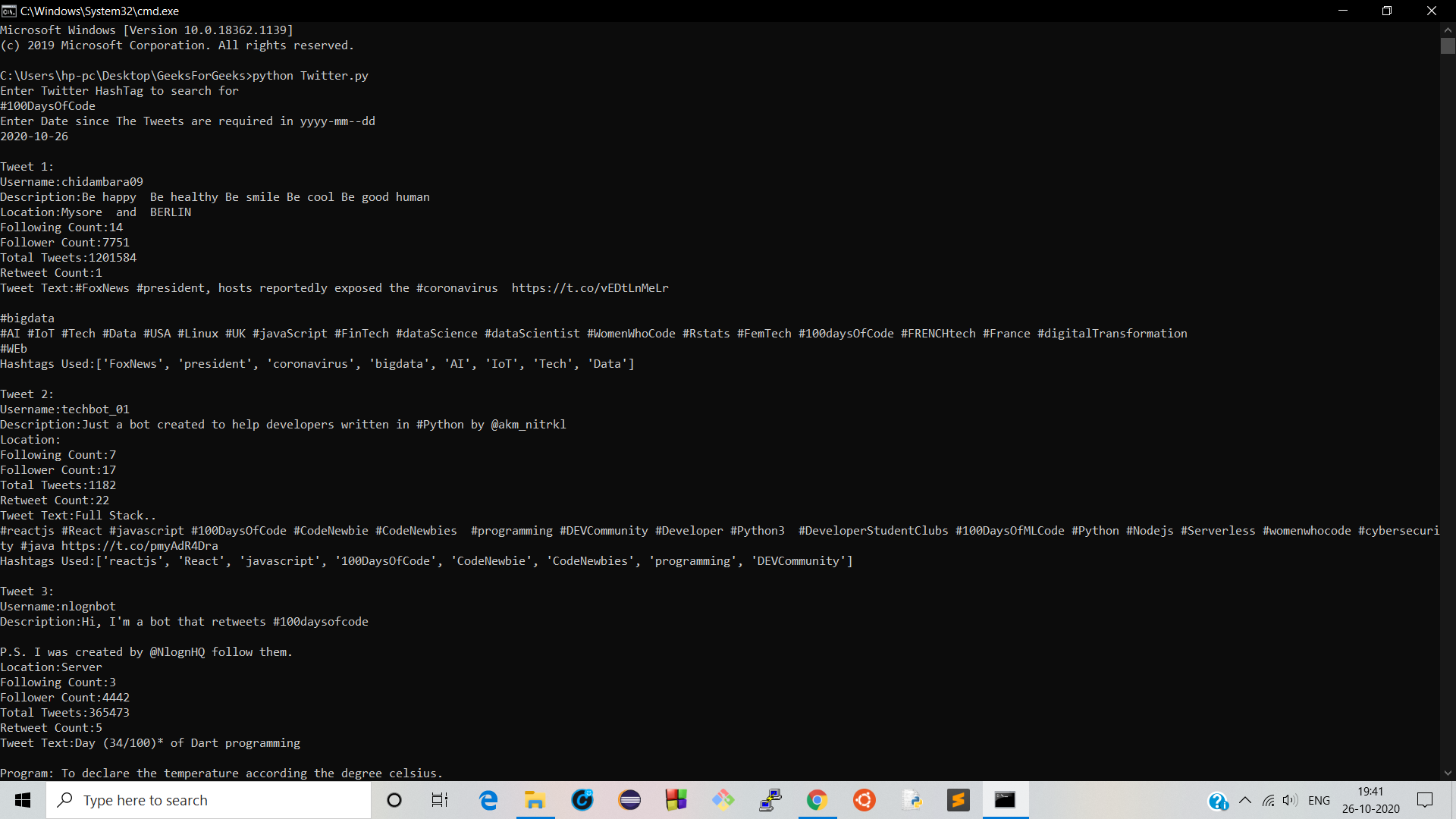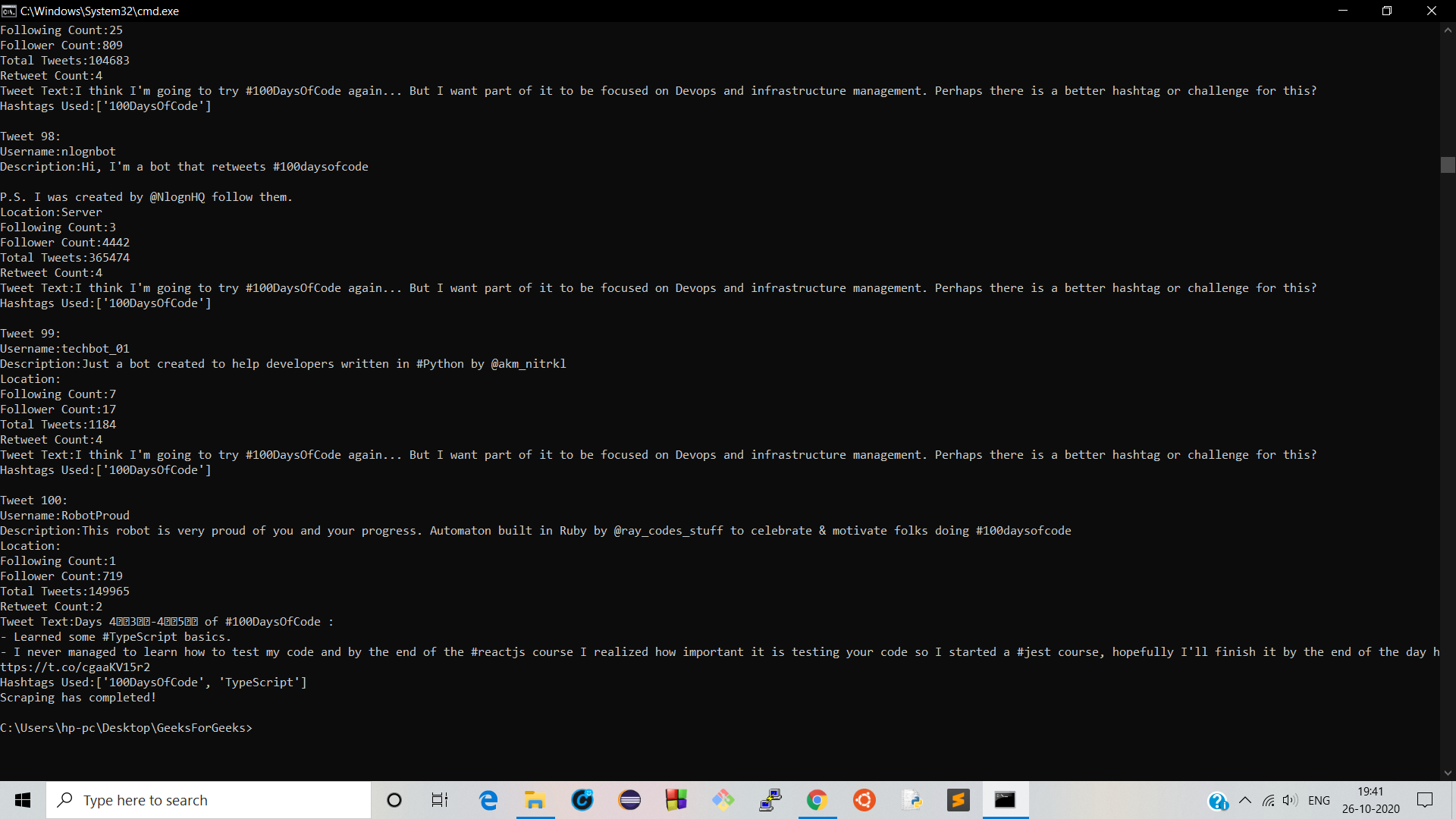# Extracting Tweets containing a particular Hashtag using Python

• Last Updated : 29 Dec, 2021

Twitter is one of the most popular social media platforms. The Twitter API provides the tools you need to contribute to, engage with, and analyze the conversation happening on Twitter, which finds a lot of application in fields like Data Analytics and Artificial Intelligence. This article focuses on how to extract tweets having a particular Hashtag starting from a given date.

### Requirements:

• Tweepy is a Python package meant for easy accessing of the Twitter API. Almost all the functionality provided by Twitter API can be used through Tweepy. To install this type the below command in the terminal.
`pip install Tweepy`
• Pandas is a very powerful framework for data analysis in python. It is built on Numpy Package and its key data structure is a DataFrame where one can manipulate tabular data. To install this type the below command in the terminal.
`pip install pandas`

### Prerequisites:

• Create a Twitter Developer account and obtain your consumer secret key and access token
• Install Tweepy and Pandas module on your system by running this command in Command Prompt

### Step-by-step Approach:

1. Import required modules.
2. Create an explicit function to display tweet data.
3. Create another function to scrape data regarding a given Hashtag using tweepy module.
4. In the Driver Code assign Twitter Developer account credentials along with the Hashtag, initial date and number of tweets.
5. Finally, call the function to scrape the data with Hashtag, initial date and number of tweets as argument.

Below is the complete program based on the above approach:

## Python

 `# Python Script to Extract tweets of a``# particular Hashtag using Tweepy and Pandas` `# import modules``import` `pandas as pd``import` `tweepy` `# function to display data of each tweet``def` `printtweetdata(n, ith_tweet):``        ``print``()``        ``print``(f``"Tweet {n}:"``)``        ``print``(f``"Username:{ith_tweet}"``)``        ``print``(f``"Description:{ith_tweet}"``)``        ``print``(f``"Location:{ith_tweet}"``)``        ``print``(f``"Following Count:{ith_tweet}"``)``        ``print``(f``"Follower Count:{ith_tweet}"``)``        ``print``(f``"Total Tweets:{ith_tweet}"``)``        ``print``(f``"Retweet Count:{ith_tweet}"``)``        ``print``(f``"Tweet Text:{ith_tweet}"``)``        ``print``(f``"Hashtags Used:{ith_tweet}"``)`  `# function to perform data extraction``def` `scrape(words, date_since, numtweet):` `        ``# Creating DataFrame using pandas``        ``db ``=` `pd.DataFrame(columns``=``[``'username'``,``                                   ``'description'``,``                                   ``'location'``,``                                   ``'following'``,``                                   ``'followers'``,``                                   ``'totaltweets'``,``                                   ``'retweetcount'``,``                                   ``'text'``,``                                   ``'hashtags'``])` `        ``# We are using .Cursor() to search``        ``# through twitter for the required tweets.``        ``# The number of tweets can be``        ``# restricted using .items(number of tweets)``        ``tweets ``=` `tweepy.Cursor(api.search_tweets,``                               ``words, lang``=``"en"``,``                               ``since_id``=``date_since,``                               ``tweet_mode``=``'extended'``).items(numtweet)`  `        ``# .Cursor() returns an iterable object. Each item in``        ``# the iterator has various attributes``        ``# that you can access to``        ``# get information about each tweet``        ``list_tweets ``=` `[tweet ``for` `tweet ``in` `tweets]` `        ``# Counter to maintain Tweet Count``        ``i ``=` `1` `        ``# we will iterate over each tweet in the``        ``# list for extracting information about each tweet``        ``for` `tweet ``in` `list_tweets:``                ``username ``=` `tweet.user.screen_name``                ``description ``=` `tweet.user.description``                ``location ``=` `tweet.user.location``                ``following ``=` `tweet.user.friends_count``                ``followers ``=` `tweet.user.followers_count``                ``totaltweets ``=` `tweet.user.statuses_count``                ``retweetcount ``=` `tweet.retweet_count``                ``hashtags ``=` `tweet.entities[``'hashtags'``]` `                ``# Retweets can be distinguished by``                ``# a retweeted_status attribute,``                ``# in case it is an invalid reference,``                ``# except block will be executed``                ``try``:``                        ``text ``=` `tweet.retweeted_status.full_text``                ``except` `AttributeError:``                        ``text ``=` `tweet.full_text``                ``hashtext ``=` `list``()``                ``for` `j ``in` `range``(``0``, ``len``(hashtags)):``                        ``hashtext.append(hashtags[j][``'text'``])` `                ``# Here we are appending all the``                ``# extracted information in the DataFrame``                ``ith_tweet ``=` `[username, description,``                             ``location, following,``                             ``followers, totaltweets,``                             ``retweetcount, text, hashtext]``                ``db.loc[``len``(db)] ``=` `ith_tweet` `                ``# Function call to print tweet data on screen``                ``printtweetdata(i, ith_tweet)``                ``i ``=` `i``+``1``        ``filename ``=` `'scraped_tweets.csv'` `        ``# we will save our database as a CSV file.``        ``db.to_csv(filename)` `if` `__name__ ``=``=` `'__main__'``:` `        ``# Enter your own credentials obtained``        ``# from your developer account``        ``consumer_key ``=` `"XXXXXXXXXXXXXXXXXXXXX"``        ``consumer_secret ``=` `"XXXXXXXXXXXXXXXXXXXXX"``        ``access_key ``=` `"XXXXXXXXXXXXXXXXXXXXX"``        ``access_secret ``=` `"XXXXXXXXXXXXXXXXXXXXX"`  `        ``auth ``=` `tweepy.OAuthHandler(consumer_key, consumer_secret)``        ``auth.set_access_token(access_key, access_secret)``        ``api ``=` `tweepy.API(auth)` `        ``# Enter Hashtag and initial date``        ``print``(``"Enter Twitter HashTag to search for"``)``        ``words ``=` `input``()``        ``print``(``"Enter Date since The Tweets are required in yyyy-mm--dd"``)``        ``date_since ``=` `input``()` `        ``# number of tweets you want to extract in one run``        ``numtweet ``=` `100``        ``scrape(words, date_since, numtweet)``        ``print``(``'Scraping has completed!'``)`

Output:Demo:

My Personal Notes arrow_drop_up